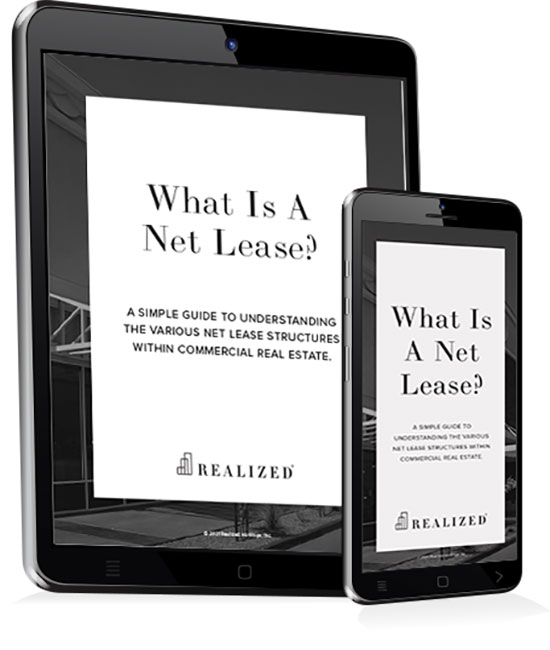Variable operating expenses are the actual costs associated with operating a property that vary in relation to a property’s occupancy rate or volume of some activity. Utilities are an example of a variable operating cost.

Other variable expenses include property management, apartment preparations for new tenants, and maintenance. As occupancy changes, the previously mentioned expenses will change. The two should have a close correlation.

We can find the expenses of a real estate operation by subtracting net income from gross income. If, during a month, we have gross income of \$10,000 and net income of \$3,500, our expenses are \$7,500. Within those expenses are a mixture of fixed and variable. Breaking out fixed vs. variable expenses allows us to better analyze these expenses.

For example, let’s say that \$4,000 is variable, and \$3,500 is fixed. We can then determine the operations expense ratios:

Variable expense: 4,000/10,000 = 40%

Fixed expenses: 3500/10,000 = 35%

What does this tell us? As income increases or decreases, our expenses will adjust. Variable expenses should maintain within the vicinity of 40% of gross income. Fixed expenses will remain fixed at around \$3,500. Variable expenses will fluctuate on a percentage basis, while fixed expenses will remain static on a dollar basis. This information can be used to help with budgeting and creating financial projections.

By keeping track of the variable expense to gross income ratio, a landlord can determine if variable expenses are increasing above their historical rate. If they are, management should find out why. There are likely some efficiencies occurring within the operation. Detecting these efficiencies through performance metric tracking, such as the variable expense to gross income ratio, provides a way for management to adjust costs before they become a bigger problem.

### The Investor's Guidebook To Net Lease